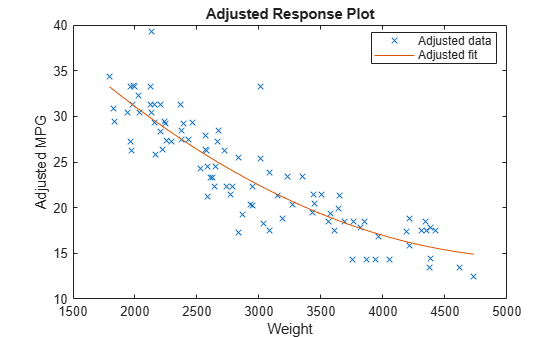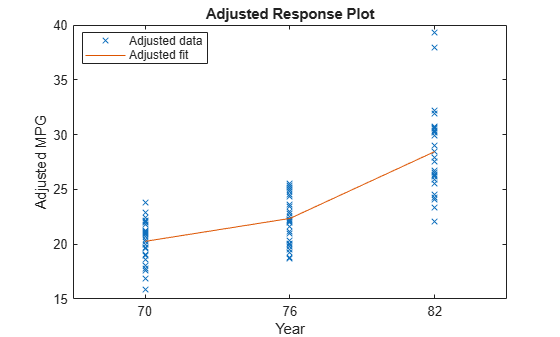Adjusted response plot of linear regression model

Description

example

plotAdjustedResponse(mdl,var) creates an adjusted response plot for the variable var in the linear regression model mdl.

plotAdjustedResponse(mdl,var,Name,Value) specifies graphical properties of adjusted response data points using one or more name-value pair arguments. For example, you can specify the marker symbol and size for the data points.

h = plotAdjustedResponse(___) returns line objects using any of the input argument combinations in the previous syntaxes. Use h to modify the properties of a specific line after you create the plot. For a list of properties, see Line Properties.

Examples

collapse all

Load the carsmall data set and fit a linear model of the mileage as a function of model year, weight, and weight squared.

tbl = table(MPG,Weight);
tbl.Year = categorical(Model_Year);
mdl = fitlm(tbl,'MPG ~ Year + Weight^2');

Plot the effect of Weight averaged over Year.Plot the effect of Year averaged over Weight.Input Arguments

collapse all

Linear regression model, specified as a LinearModel object created using fitlm or stepwiselm.

Variable for the adjusted response plot, specified as a character vector or string array of the variable name in mdl.VariableNames, or a positive integer representing the index of a variable in mdl.VariableNames.

Data Types: char | string | single | double

Name-Value Arguments

Specify optional comma-separated pairs of Name,Value arguments. Name is the argument name and Value is the corresponding value. Name must appear inside quotes. You can specify several name and value pair arguments in any order as Name1,Value1,...,NameN,ValueN.

Example: 'Color','blue','Marker','*'

Note

The graphical properties listed here are only a subset. For a complete list, see Line Properties. The specified properties determine the appearance of adjusted response data points.

Line color, specified as the comma-separated pair consisting of 'Color' and an RGB triplet, hexadecimal color code, color name, or short name for one of the color options listed in the following table.

The 'Color' name-value pair argument also determines marker outline color and marker fill color if 'MarkerEdgeColor' is 'auto' (default) and 'MarkerFaceColor' is 'auto'.

For a custom color, specify an RGB triplet or a hexadecimal color code.

• An RGB triplet is a three-element row vector whose elements specify the intensities of the red, green, and blue components of the color. The intensities must be in the range [0,1]; for example, [0.4 0.6 0.7].

• A hexadecimal color code is a character vector or a string scalar that starts with a hash symbol (#) followed by three or six hexadecimal digits, which can range from 0 to F. The values are not case sensitive. Thus, the color codes '#FF8800', '#ff8800', '#F80', and '#f80' are equivalent.

Alternatively, you can specify some common colors by name. This table lists the named color options, the equivalent RGB triplets, and hexadecimal color codes.

Color NameShort NameRGB TripletHexadecimal Color CodeAppearance
'red''r'[1 0 0]'#FF0000''green''g'[0 1 0]'#00FF00''blue''b'[0 0 1]'#0000FF''cyan' 'c'[0 1 1]'#00FFFF''magenta''m'[1 0 1]'#FF00FF''yellow''y'[1 1 0]'#FFFF00''black''k'[0 0 0]'#000000''white''w'[1 1 1]'#FFFFFF''none'Not applicableNot applicableNot applicableNo color

Here are the RGB triplets and hexadecimal color codes for the default colors MATLAB® uses in many types of plots.

[0 0.4470 0.7410]'#0072BD'[0.8500 0.3250 0.0980]'#D95319'[0.9290 0.6940 0.1250]'#EDB120'[0.4940 0.1840 0.5560]'#7E2F8E'[0.4660 0.6740 0.1880]'#77AC30'[0.3010 0.7450 0.9330]'#4DBEEE'[0.6350 0.0780 0.1840]'#A2142F'Example: 'Color','blue'

Line width, specified as the comma-separated pair consisting of 'LineWidth' and a positive value in points. If the line has markers, then the line width also affects the marker edges.

Example: 'LineWidth',0.75

Marker symbol, specified as the comma-separated pair consisting of 'Marker' and one of the values in this table.

MarkerDescriptionResulting Marker
'o'Circle'+'Plus sign'*'Asterisk'.'Point'x'Cross'_'Horizontal line'|'Vertical line's'Square'd'Diamond'^'Upward-pointing triangle'v'Downward-pointing triangle'>'Right-pointing triangle'<'Left-pointing triangle'p'Pentagram'h'Hexagram'none'No markersNot applicable

Example: 'Marker','+'

Marker outline color, specified as the comma-separated pair consisting of 'MarkerEdgeColor' and an RGB triplet, hexadecimal color code, color name, or short name for one of the color options listed in the Color name-value pair argument.

The default value of 'auto' uses the same color specified by using 'Color'.

Example: 'MarkerEdgeColor','blue'

Marker fill color, specified as the comma-separated pair consisting of 'MarkerFaceColor' and an RGB triplet, hexadecimal color code, color name, or short name for one of the color options listed in the Color name-value pair argument.

The 'auto' value uses the same color specified by using 'Color'.

Example: 'MarkerFaceColor','blue'

Marker size, specified as the comma-separated pair consisting of 'MarkerSize' and a positive value in points.

Example: 'MarkerSize',2

Output Arguments

collapse all

Line objects, returned as a 2-by-1 vector. h(1) corresponds to the adjusted response data points, and h(2) corresponds to the adjusted response function. Use dot notation to query and set properties of the line objects. For details, see Line Properties.

You can use name-value pair arguments to specify the appearance of adjusted response data points corresponding to the first graphics object h(1).

collapse all

An adjusted response function describes the relationship between the fitted response and a single predictor, with the other predictors averaged out by averaging the fitted values over the data used in the fit.

A regression model for the predictor variables (x1, x2, …, xp) and the response variable y has the form

yi = f(x1i, x2i, …, xpi) + ri,

where f is a fitted regression function and r is a residual. The subscript i represents the observation number.

The adjusted response function for the first predictor variable x1, for example, is defined as

$g\left({x}_{1}\right)=\frac{1}{n}\sum _{i=1}^{n}f\left({x}_{1},{x}_{2i},{x}_{3i},...,{x}_{pi}\right),$

where n is the number of observations. The adjusted response data value is the sum of the adjusted fitted value and the residual for each observation.

${\stackrel{˜}{y}}_{i}=g\left({x}_{1i}\right)+{r}_{i}.$

plotAdjustedResponse plots the adjusted response function and the adjusted response data values for a selected predictor variable.

Tips

• The data cursor displays the values of the selected plot point in a data tip (small text box located next to the data point). The data tip includes the x-axis and y-axis values for the selected point, along with the observation name or number.

Alternative Functionality

• A LinearModel object provides multiple plotting functions.

• When creating a model, use plotAdded to understand the effect of adding or removing a predictor variable.

• When verifying a model, use plotDiagnostics to find questionable data and to understand the effect of each observation. Also, use plotResiduals to analyze the residuals of the model.

• After fitting a model, use plotAdjustedResponse, plotPartialDependence, and plotEffects to understand the effect of a particular predictor. Use plotInteraction to understand the interaction effect between two predictors. Also, use plotSlice to plot slices through the prediction surface.

• plotPartialDependence creates either a line plot or a surface plot of predicted responses against a single feature or a pair of features, respectively, by marginalizing over the other variables. A line plot for a single feature from plotPartialDependence and an adjusted response function plot from plotAdjustedResponse are the same within numerical precision.

• plotEffects creates a summary plot that shows separate effects for all predictors.

• plotAdded shows the incremental effect on the response of specified terms by removing the effects of the other terms, whereas plotAdjustedResponse shows the effect of a selected predictor in the model fit with the other predictors averaged out by averaging the fitted values. Note that the definitions of adjusted values in plotAdded and plotAdjustedResponse are not the same.